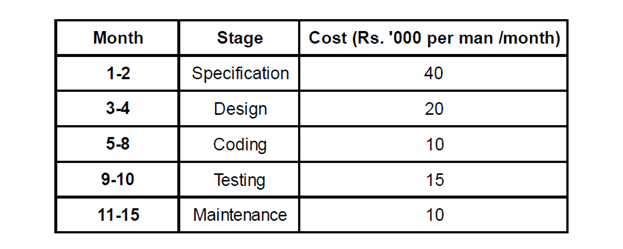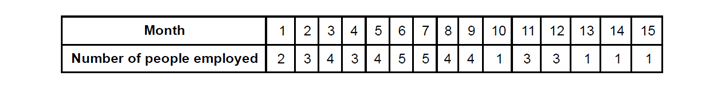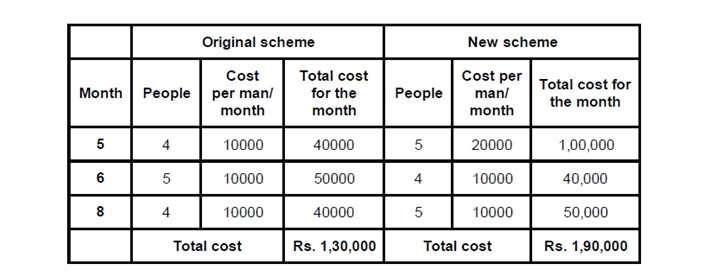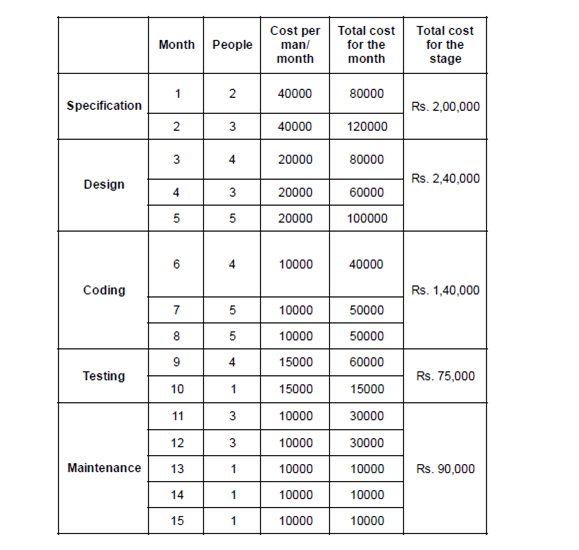Direction for questions 1 to 5: Answer the questions based on the following information. Chrome Software Co., before selling a package to its clients, follows the given schedule.The number of people employed in each month is:Question 1. Due to overrun in ‘design’, the design stage took 3 months, i.e. months 3, 4 and 5. The number of people working on design in the fifth month was 5. Calculate the percentage change in the cost incurred in the fifth month. (Due to improvement in ‘coding’ technique, this stage was completed in months 6-8 only.)
a. 225%
b. 150%
c. 275%
d. 240%

Question 2. With reference to the above question, what is the cost incurred in the new ‘coding’ stage? (Under thenew technique, 4 people work in the sixth month and 5 in the eighth.)
a. Rs. 1,40,000
b. Rs. 1,50,000
c. Rs. 1,60,000
d. Rs. 1,70,000

Question 3. What is the difference in cost between the old and the new techniques?
a. Rs. 30,000
b. Rs. 60,000
c. Rs. 70,000
d. Rs. 40,000

Question 4. Under the new technique, which stage of software development is most expensive for Chrome SoftwareCo.?
a. Testing
b. Specification
c. Coding
d. Design

Question 5. Which five consecutive months have the lowest average cost per man-month under the new technique?
a. 1-5
b. 9-13
c. 11-15
d. None of these

From the given data we know that originally for the 5th month, 4 people were scheduled to do coding. Total cost for 4 people = (10000 × 4) = Rs. 40,000.

Now there are 5 people who are working on design in the 5th month.

Total cost for 5 people = (20000 × 5) = Rs.1,00,000.

∴percentage change in the cost incurred in the fifth month =  {(100000 – 40000)/40000} x 100 = 150%

On the basis of the previous question, we know that that the coding stage is now completed in 6th, 7th and 8th months.

In the 6th month,  4 people are employed  and in the 8th month 5 people are employed.

In the 7th month also, there are 5 people employed (from previous data).

After combining these months we find that the total cost incurred in the coding stage = (5 + 5 + 4) × 10000 = Rs.1,40,000.

The difference in the cost will arise only in 5th , 6th and 8th month. Comparison of the costs is given below in the table:From the above table we can conclude that the difference in the cost between the old and the new technique is Rs. 60,000.

We can determine which phase was the most costly by calculating the cost incurred in various stages under the present scheme as given below in the table: